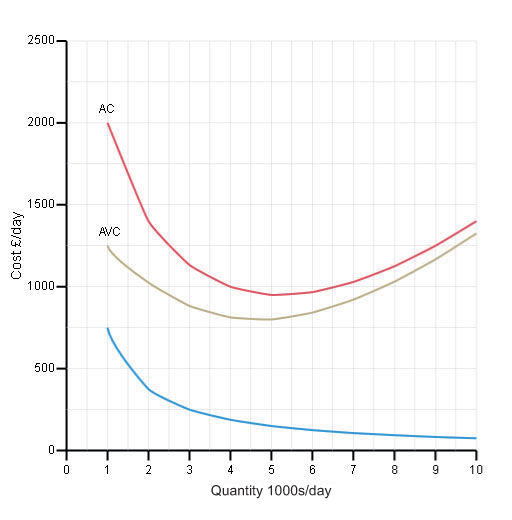Economics and the 2008 crisis: a Keynesian view

Start this free course now. Just create an account and sign in. Enrol and complete the course for a free statement of participation or digital badge if available.

Short run cost curves (4)

Activity 23c

If output is at a quantity of 3000 per day, using the figure below, pick one answer describing how the average cost will change if output is increased to 3001 per day.Figure 41 Average cost curves (4)

a.

Increase

b.

Decrease

c.

Remain unchanged

If output increases from a quantity of 3000 per day, average cost will fall. This is because at this output the average cost curve is sloping downwards.

Increasing output will reduce the average cost until it reaches a minimum at a quantity of approximately 5000 per day. Then the average cost curve slopes upwards, so average cost increases when output increases beyond 5000 per day.